# 新型コロナウィルス（COVID-19）環境下における保育施設の利用状況の分析

#### はじめに

こんにちは。研究開発部の島田です。今回は新型コロナウィルス（COVID-19）環境下における全国の保育施設への影響について、ICTサービスキッズリーの連絡帳機能のデータを使って調査・分析を行いましたので、その結果をご報告いたします。

#### 分析条件

キッズリーに登園または欠席情報どちらも入力されなかったユーザーのデータは分析には含まれていません。

#### 10都道府県における園児の登園状況

1. 2020年3月23日〜3月27日
2. 2020年4月13日〜4月17日
3. 2020年5月11日〜5月15日
4. 2020年5月25日〜5月29日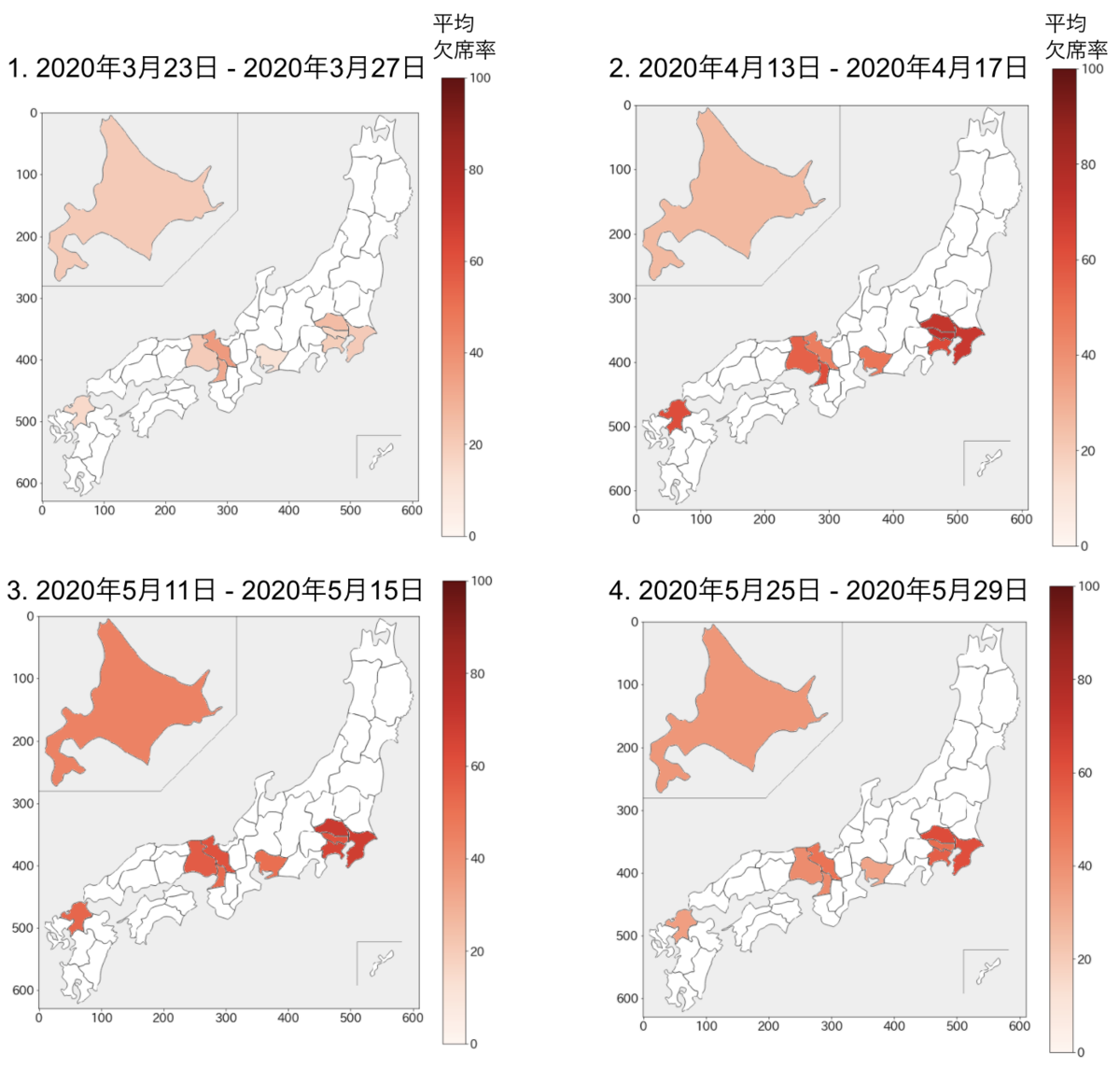図1 : 10都道府県における保育施設の園児の平均欠席率

また、直近の5月25日の週（期間4）においては、平均欠席率が関東でも60%程度まで下がってきており、緊急事態宣言が解除されたことによって、少しずつ登園する園児が増えてきていることが見てとれます。

#### 10都道府県における緊急事態宣言期間の保育施設の稼働状況

なお、今回の調査における保育施設の稼働率の定義については、対象期間内に1人以上の園児が登園された場合を稼働しているとし、登園園児数が0人だった場合を未稼働としました。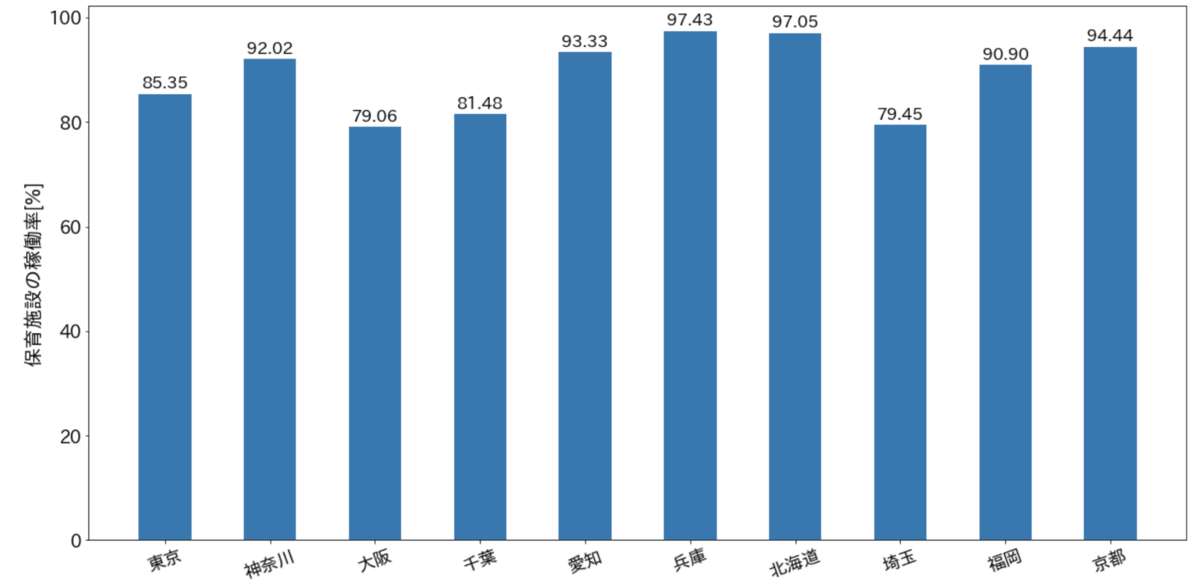図2 : 10都道府県における緊急事態宣言期間の保育施設の稼働率

#### 欠席コメント欄への記入率の推移

キッズリーでは園児が欠席する際には保護者がコメントを残せる機能があり、そのコメント欄への1日ごとの記入率を算出しました。なお、本調査では全国の保育施設のデータを対象とし、分析対象期間は2020年2月1日〜4月30日としました。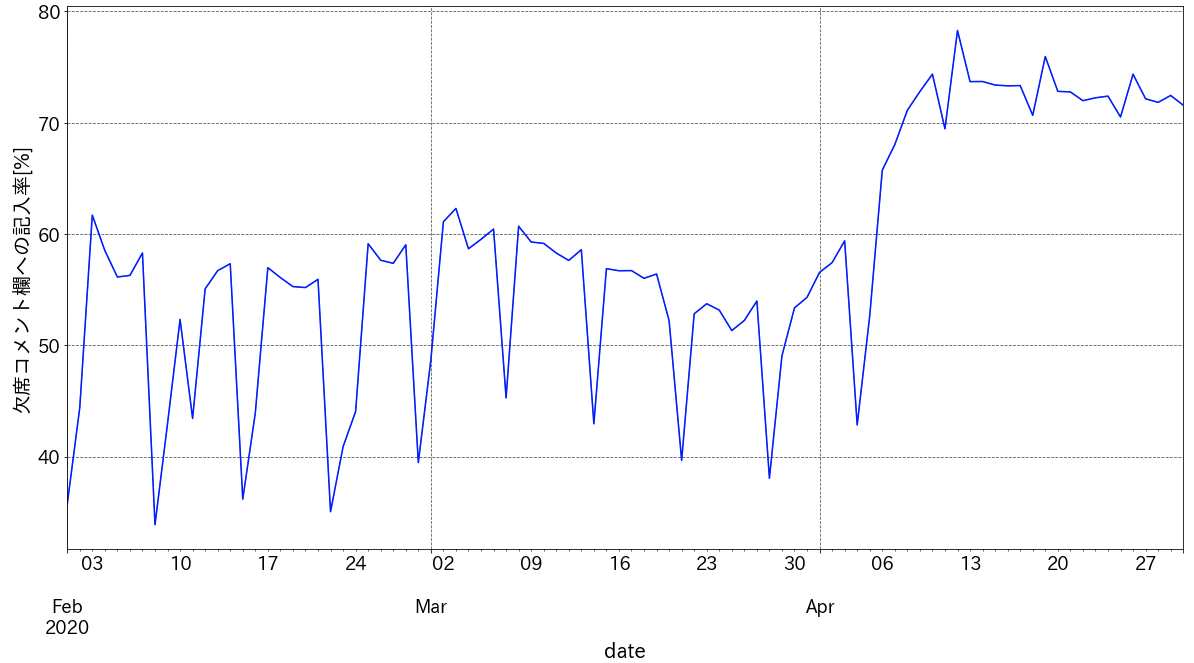図3 : 欠席コメント欄への記入率

2020年4月7日以前では、平日における欠席コメント欄への記入率はおよそ55％前後で推移していますが、緊急事態宣言が発令された2020年4月7日以降では欠席コメント欄への記入率がおよそ75％程度で推移しており、これまで以上にキッズリーの連絡機能を使って園と保護者間でのコミュニケーションツールとして活用されていることがわかります。

#### 欠席コメント欄の頻出単語を可視化

1. 2020年2月1日〜2020年2月29日
2. 2020年4月1日〜2020年4月30日

2020年2月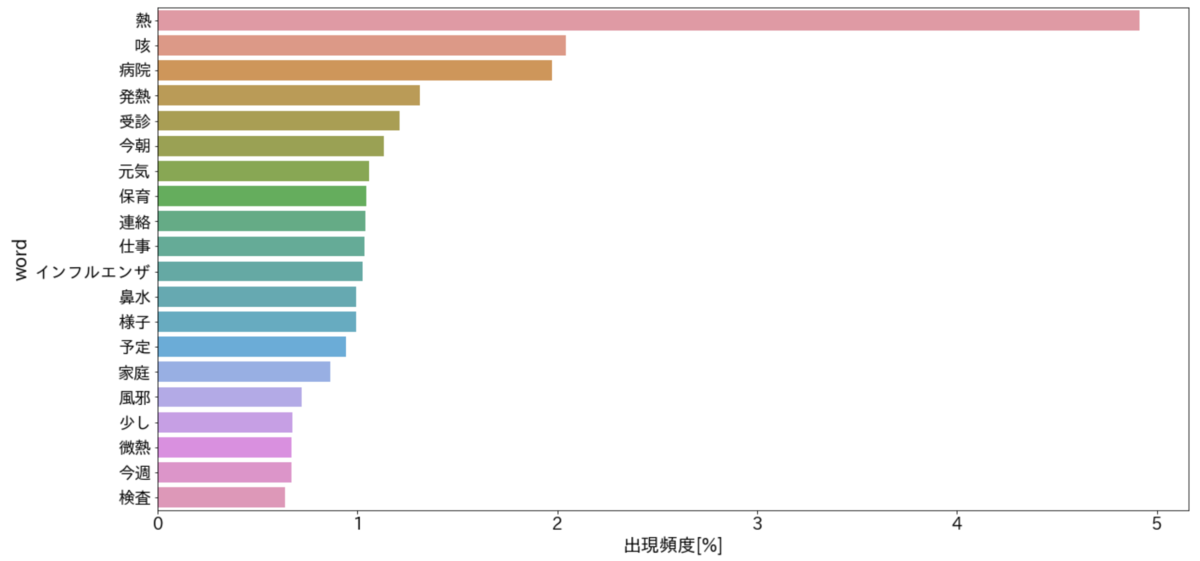2020年4月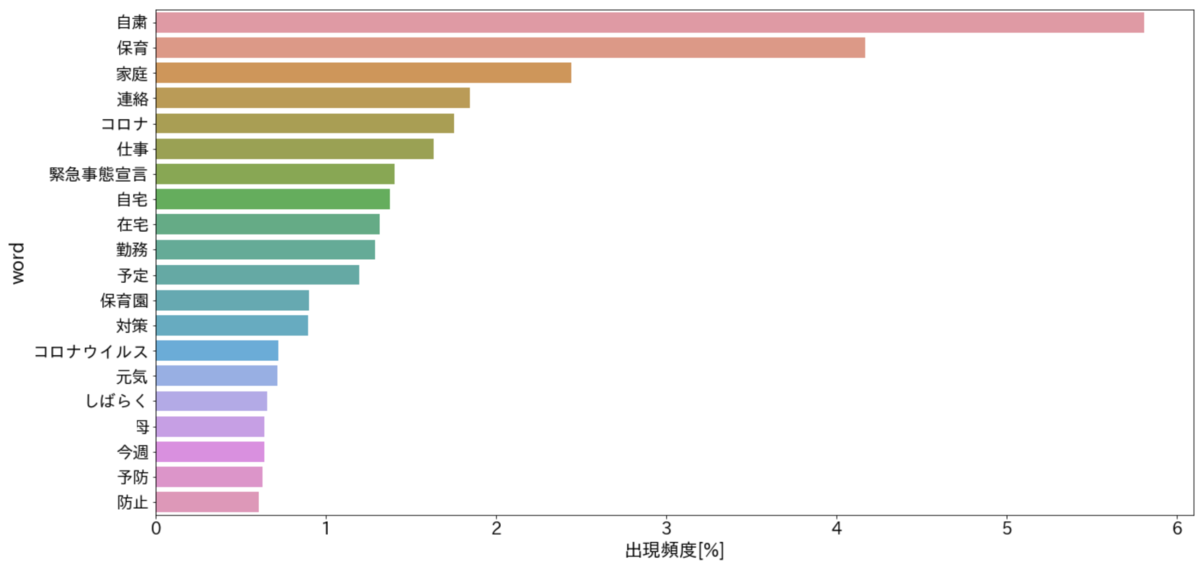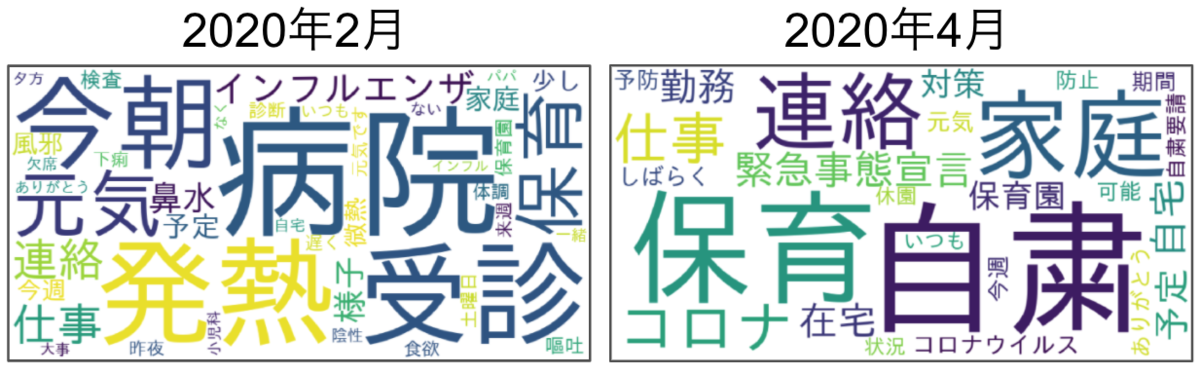図5 : 欠席コメント欄の頻出単語の可視化

#### 本データの引用について

その上で、引用される際は「ユニファ株式会社調査結果」の明記とリンクをよろしくお願いいたします。

#### 最後に

こういった状況を踏まえ、ユニファ株式会社では最前線で働く保育者をはじめとする保育現場スタッフと保育施設を応援するため、以下の二つのプロジェクトを実施中です。

# Terraform内でGithubのreleaseをダウンロードして利用する

おはようございます、こんにちは、こんばんわ ユニファでインフラみてますすずきです。

そんな中、TerraformでGithubからパッケージをダウンロードしながらLambdaにアップロードできるのか試してみたのでそれを書いてみます。

※ 今回の最終的なコードGist

# サーバ / アプリ担当者が混在するチームにおける、ベロシティ計測・リファレンスストーリーの考え方

…これ、まるで恋をしているようではありませんか？

そうなんです、私達はバーンダウンチャートに恋をしているのです。

### 本題

「サーバ / アプリ担当者が混在するチーム」と記載しましたが、要はそれぞれで専門領域があり、お互いの作業を代わることができない場合のことです。

• 本題
• 結論
• 前提
• ベロシティ計測
• パターン①：同じチームなんだし、サーバ / アプリの見積もりをまとめて表示しちゃおうよ！案
• パターン②：担当する作業ごとに分けて表示しようよ！案
• リファレンスストーリー
• まとめ
• さいごに

### 結論

はじめに結論ですが、同じチームと言えど、お互いの作業を代わることができないのであればリファレンスストーリーは別々で用意し、ベロシティ計測も異なるチームとして扱うべきです。

では何故そうすべきなのか？については、以降のセクションで、「ベロシティ計測」「リファレンスストーリー」の順に図を使いながら説明していきます。

#### 前提

なお、以降の説明では下記の前提条件を使用していきます。

• 人数
• サーバ担当：3名
• アプリ担当：2名
• 合計見積もり
• サーバ：250pt
• アプリ：50pt
• 1 sprintで一人あたり、 5pt を消化できる
• サーバ：3名 × 5pt = 15pt
• アプリ：2名 × 5pt = 10pt

# Create SlackBot using AWS API Gateway and Lambda

By Maimit Patel, Software Engineer at ユニファ

In this blog I am going to create SlackBot using AWS API Gateway, AWS Lambda and Slack Event API.

#### What is SlackBot?

A SlackBot is a regular app that is designed to interact with the user via conversation. When user mention the SlackBot app from anywhere in the slack then it will access the APIs and do all of the magical things that Slack App can do.

#### 1. Setup Slack App

• Go to the Slack apps home page and click `Create New App` button.

• Add scopes under the `OAuth & Permissions` menu

• Installing App to Workspace

• This will create Bot User, check it on `App Home` page

#### 2. Configure Docker Image to run the Lambda function in the local environment

• Pull the lambci/lambda:ruby2.7 docker image
```# Open command prompt in your local machine and
# hit this command to pull the docker image of Lambda with runtime Ruby-2.7
\$ docker pull lambci/lambda:ruby2.7```

#### 3. Create a Lambda function that responds to the Slack

• Setup application directory to store the Lambda ruby function
```# Creating directory
\$ mkdir greeting-bot

# Move to directory
\$ cd greeting-bot ```
• Create file greeting_bot.rb under greeting-bot directory
```# Creating ruby file under greeting-bot directory
\$ touch greeting_bot.rb```
• Set environment variables

• Find Bot User OAuth Access Token under the `OAuth & Permissions` menu from Slack App

``` \$ export BOT_OAUTH_TOKEN='bot_oauth_token' ```

• Find Verification Token under `Basic Information` menu from Slack App

``` \$ export VERIFICATION_TOKEN='verification_token' ```

• Update the file greeting_bot.rb

```class GreetingBot
def self.main(event:, context:)
new.run(event)
end

def run(event)
case event['type']
when 'url_verification'
verify(event['token'], event['challenge'])
when 'event_callback'
if event['event']['type'] == 'app_mention'
process(event['event']['text'], event['event']['channel'])
end
end
end

private

# Verify request from the slack
def verify(token, challenge)
if token == ENV['VERIFICATION_TOKEN']
{ body: { challenge: challenge } }
else
{ body: 'Invalid token' }
end
end

def process(text, channel)
body = if text.strip.downcase.include?('hello')
'Hi, How are you?'
else
end
send_message(body, channel)
end

# Slack API response to the mentioned channel
def send_message(text, channel)
uri = URI('https://slack.com/api/chat.postMessage')
params = {
token: ENV['BOT_OAUTH_TOKEN'],
text: text,
channel: channel
}
uri.query = URI.encode_www_form(params)

Net::HTTP.get_response(uri)
end
end```
• Run this Lambda function in the local environment and response back to the mentioned channel from request
```# Make sure the mentioned channel(i.e greeting-channel) has already invited the greeting-bot that we have created in step 1

docker run \
-e BOT_OAUTH_TOKEN=\$BOT_OAUTH_TOKEN \
greeting_bot.GreetingBot.main \
'{"type": "event_callback","event":{"type":"app_mention","text":"<@U>hello!","channel":"greeting-channel"}}'```
• Result in local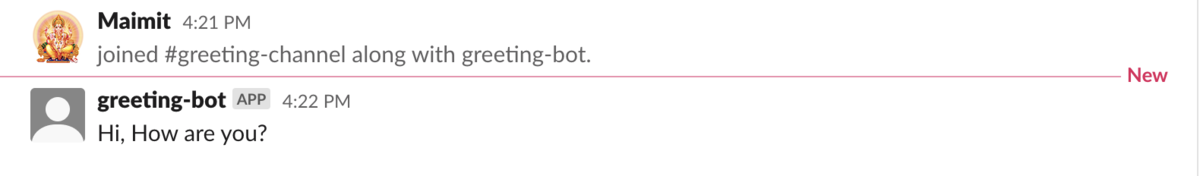#### 4. Upload Lambda function to the AWS Lambda

• Make sure you have created a Lambda function in AWS.

• Upload Lambda function from the local machine using AWS CLI

```# Creating zip file
zip function.zip greeting_bot.rb

# Upload zip file to the Lambda
\$ aws lambda update-function-code --function-name slack-greeting-bot --zip-file fileb://function.zip
# add `--profile profile_name` if you got the AccessDeniedException```
• Set environment variables BOT_OAUTH_TOKEN and VERIFICATION_TOKEN in Lambda

• After upload to Lambda, it looks like this

#### 5. Create REST API end-point using API Gateway that calls the lambda function

• I have created API Gateway

• How to create REST API using API Gateway? read more
• Add trigger to Lambda function

• Click the `Add trigger` button from the designer pane of the Lambda function
• Select the trigger(i.e API Gateway) from options
• Select the REST API created in the `step 5`

#### 6. Set the API Gateway end-point to Slack Event Request URL

• Go to `Event Subscriptions` menu from the Slack App and enable the event subscription
• Enter and Verify the Request URL on same page
• Request URL is API Gateway end-point that we have done in `step 5`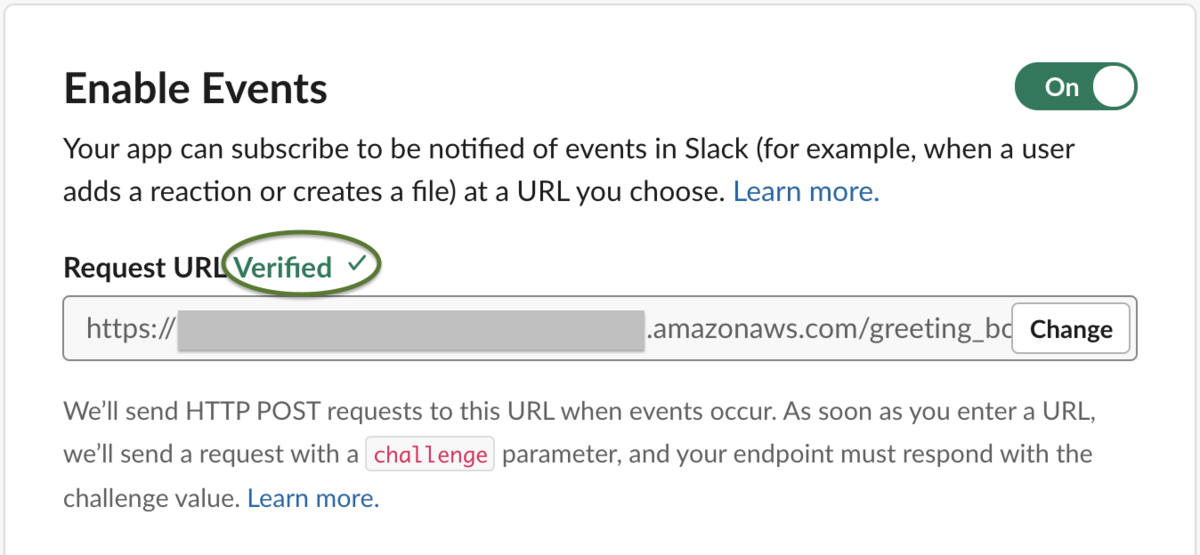Enable event subscription & Verify RESR API end-point

#### 7. Test the SlackBot by calling from #greeting-channel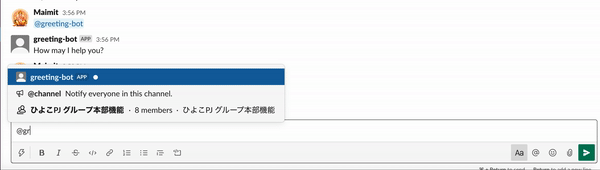# 新型コロナウイルスと多国籍チーム

みなさんこんばんは。

ユニファでエンジニアのマネージャーをしている田渕です。

これまでユニファの開発者ブログでも、何回かに渡り新型コロナウイルスに端を発した 在宅勤務に関する記事やインフラ関連の記事などを公開してきましたが、 今回は新型コロナウイルス による非日常をマネージャー視点で見たときの気付きについて書いてみました。

### まずは前提

• 現在、私を除くと全部で17名のエンジニアが居ます。

• そのうち、国籍が日本ではない方は、12名です。

• 参考までに国名を記載しておくと、中国、韓国、台湾、フィリピン、インド、バングラデシュ、スペイン、イギリス、ポーランド、ロシアです。

• 他の記事でも触れられているように、現在の勤務は基本的に在宅勤務です。

### 不穏な空気が流れはじめた1月末

この数ヶ月、色々なことがあり、なんだか既にとても遠い昔のような感覚ですが。。。 日本国内で「新型コロナウイルス 」の話が本格的に聞かれるようになったのは、1月下旬くらいだったでしょうか。 その頃、まだ日本人はどこか対岸の火事のような感覚だった方が大半ではないかと思います。

### この環境下でやってきたこと

#### なるべく孤立を防ぐ

##### 背景

メンバーの中には「ユニファに入社するために初めて日本に来た」と言う方もいます。（ありがたいことです。） そのような慣れない環境の中で経験したことのないパンデミック、しかも自粛で部屋に籠もらなければならないと言うのは、なかなかに厳しい状況でした。 やはりメンバーからは「寂しい」と言った声を聞くようになりました。

### さいごに

ユニファでは一緒に働いてくれる仲間を募集中です！

yoshiori さん同様に、これによってみんなに何かを強制するものではないですし、自分としてはこうありたいと思うことを書いていますがまだまだ不十分なところも多いです。

# My Role

では私は何をする人なのかというと、そういったプロ集団がチームとしてより良いアウトプットを出すにはどうすれば良いのかを考え、環境や体制をつくり、アウトプットを最大化するためのことをする人です。また、良いプロダクトを作るという視点に、経営としてのビジネスの視点を加え、チームが目指す方向を考えます。ユニファには VPoE がいないこともあり、要素としては技術面よりもメンバーのマネジメントやチームアップなど、 VPoE の役割にあたる要素が多いと思います。また、クリエイティブなことやドキュメント作成は苦手分野で、ビジネス領域には疎いので、そういった部分はみんなに助けてもらいたいと思ってます。

みんながいないとプロダクトは作れないので、力を貸してください。

# 市場価値を上げたい

もちろんみんなとはずっとユニファで一緒に仕事をしていきたいと思っていますが、この業界では転職も珍しいことではないですし、いずれはユニファを離れていく人も多いかと思います。そうなったときに、ユニファでやってきたことが無駄ではなく、それぞれ個人としての市場価値を上げて次に移れるようになっていて欲しいと思っています。そのためには極力やりたいことやスキルアップにつながるような仕事のアサインをしたいと思っていますし、みんなにも向上心を持って取り組んで欲しいと思っています。

# リスペクトを欠いた言動は嫌います

ユニファのようなスタートアップに来る人は部署問わずみんな頑張れる人ですし頑張って当たり前です。面接でもそういう人を採っているつもりですので、みんな最大限頑張ってくれていると信じてます。頑張った結果、結果が伴わないことや、進め方が良くなかったこと、他の領域のプロからしたらセオリーを無視した形になることもあり得ます。それに対しての嘲笑やリスペクトを欠いたネガティブな発言やslack等での書き込みは問題視します。表面的な結果だけでなく、その裏にある事情も想像しましょう。もちろん建設的な議論やフィードバックは大歓迎です。

# その他やってること

• システム開発本部予算検討

• 毎年の予算計画でシステム開発本部として必要な予算（プロダクトのインフラ費用や開発環境、外部への委託費用などなど）を検討し、会社全体の予算計画の中ですり合わせをします。
• 技術ブランディング

• ユニファ開発チームがどんなことをやっているか、どんなメンバーがいるかを外向けに露出する機会を増やし、技術的な信頼度の向上や、採用のためのブランディング活動をしています。
• 採用関連

• 人材紹介会社の方にどんな人材を求めているかを説明して打ち合わせしたり、応募者の書類選考、面接、オファー面談などを行っています。
• 社内インフラタスクサポート

• 社内インフラチームは1人しかいないので、対応可能なタスクはサポートしています。
• データ基盤構築での技術検討など

• データ基盤を構築していくためのアーキテクチャ検討にあたって GCP や AWS において何を使っていくのが良いのかの検討を行っています。

# パーソナリティー

• 内向的&人見知りで自分から話すのは苦手なのでパーティー等ではぼっちになりがちですが、話しかけられるのは大歓迎です。
• 気弱ですがそのくせ時々勢いで色々始めてみたりします。
• O型なので大雑把で楽観的です。

# これは常にWIPです

この内容は 2020/05/02 現在のスナップショットなので、役割や考え方が変われば今後内容の変更や大幅な修正も大いにあり得ます。

# Auto-encoder to Neural Network Learning in Pytorch

By Matthew Millar R&D Scientist at ユニファ

### Purpose:

This blog will cover a method for combining unsupervised learning with supervised learning. I will show how to use an autoencoder and combine that with a neural network for a classification problem in Pytorch.

### Data Processing:

The first step will be easy as the same dataloader can be used for both training the autoencoder and the neural network.
I will be using the cifar10 dataset as this is available to everyone and is easy to deal with.

```#Basic Transforms
SIZE = (32,32) # Resize the image to this shape
# Test and basic transform. This will reshape and then transform the raw image into a tensor for pytorch
basic = transforms.Compose([transforms.Resize(SIZE),
transforms.ToTensor()])

# Normalized transforms (0.4914, 0.4822, 0.4465), (0.247, 0.243, 0.261) retrived from here https://github.com/kuangliu/pytorch-cifar/issues/19
mean = (0.4914, 0.4822, 0.4465) # Mean
std = (0.247, 0.243, 0.261) # Standard deviation
# This will transform the image to the Size and then normalize the image
norm_tran = transforms.Compose([transforms.Resize(SIZE),
transforms.ToTensor(),
transforms.Normalize(mean=mean, std=std)])

#Simple Data Augmentation
# Data augmentations
'''
Randomly flip the images both virtically and horizontally this will cover and orientation for images
Randomly rotate the image by 15. This will give images even more orientation than before but with limiting the black board issue of rotations
Random Resie and crop this will resize the image and remove any excess to act like a zoom feature
Normalize each image and make it a tensor
'''
aug_tran = transforms.Compose([transforms.RandomHorizontalFlip(),
transforms.RandomRotation(15),
transforms.RandomResizedCrop(SIZE, scale=(0.08, 1.0), ratio=(0.75, 1.3333333333333333), interpolation=3),
transforms.ToTensor(),
transforms.Normalize(mean=mean, std=std)])

# Create Dataset
train_dataset = datasets.ImageFolder(TRAIN_DIR, transform=aug_tran)
test_dataset  = datasets.ImageFolder(TEST_DIR, transform=norm_tran) #No augmentation for testing sets
# Parameters for setting up data loaders
BATCH_SIZE = 32
NUM_WORKERS = 4
VALIDATION_SIZE = 0.15

# Validatiaon split
num_train = len(train_dataset) # Number of training samples
indices = list(range(num_train)) # Create indices for each set
np.random.shuffle(indices) # Randomlly sample each of these by shuffling
split = int(np.floor(VALIDATION_SIZE * num_train)) # Create the split for validation
train_idx , val_idx = indices[split:], indices[:split] # Create the train and validation sets
train_sampler = SubsetRandomSampler(train_idx) # Subsample using pytroch
validation_sampler = SubsetRandomSampler(val_idx) # same here but for validation

batch_size=BATCH_SIZE,
sampler=train_sampler,
num_workers=NUM_WORKERS)

batch_size=BATCH_SIZE,
sampler=validation_sampler,
num_workers=NUM_WORKERS)

batch_size=BATCH_SIZE,
shuffle=False,
num_workers=NUM_WORKERS)
```

Also, I have a list of dataloaders on my Kaggle page for both Pytorh and Keras if you would like to learn how to build out custom dataloader and datasets with both languages.
https://www.kaggle.com/matthewmillar/kerasgeneratorexamples

### Autoencoder:

An autoencoder is an unsupervised method of learning encodings of data which that can be processed efficiently. This is done through dimension reduction and ignoring noise in the dataset. There are two sides to an autoencoder. The encoder and the decoder. The encoder job is to create a useful encoding that will remove unwanted noise in the dataset while keeping the most import parts of the data. The decoder job is to take the encodings and reassemble it into the original input form. Below is the Autoencoder that we will be using as the feature extraction system in our combination model.

The approach that will be taken is to train the autoencoder separately instead of together with the NN. This will allow for us to check the result of the output of the encoder as well as the decoder and see how well it works.

```# define the NN architecture
class ConvAutoencoder(nn.Module):
def __init__(self):
super(ConvAutoencoder, self).__init__()
## encoder layers ##
# conv layer (depth from 1 --> 16), 3x3 kernels
self.conv1 = nn.Conv2d(3, 16, 3, padding=1)
# conv layer (depth from 16 --> 4), 3x3 kernels
self.conv2 = nn.Conv2d(16, 4, 3, padding=1)
# pooling layer to reduce x-y dims by two; kernel and stride of 2
self.pool = nn.MaxPool2d(2, 2)

## decoder layers ##
## a kernel of 2 and a stride of 2 will increase the spatial dims by 2
self.t_conv1 = nn.ConvTranspose2d(4, 16, 2, stride=2)
self.t_conv2 = nn.ConvTranspose2d(16, 3, 2, stride=2)

def forward(self, x):
## encode ##
# add hidden layers with relu activation function
# and maxpooling after
x = torch.relu(self.conv1(x))
x = self.pool(x)
x = torch.relu(self.conv2(x))
x = self.pool(x)  # compressed representation

## decode ##
# add transpose conv layers, with relu activation function
x = torch.relu(self.t_conv1(x))
# output layer (with sigmoid for scaling from 0 to 1)
x = torch.sigmoid(self.t_conv2(x))

return x

# Loss and optimizers
loss_function = nn.MSELoss()
scheduler = torch.optim.lr_scheduler.ReduceLROnPlateau(optimizer=optimizer, mode='min', factor=0.1, patience=3, verbose=True) # Automatically reduce learning rate on plateau

# number of epochs to train the model
n_epochs = 35
ae_model_filename = 'cifar_autoencoder.pt'
train_loss_min = np.Inf # track change in training loss

ae_train_loss_matrix = []
for epoch in range(1, n_epochs+1):
# monitor training loss
train_loss = 0.0

###################
# train the model #
###################
# _ stands in for labels, here
# no need to flatten images

images, _ = data
if use_gpu:
images = images.cuda()
# clear the gradients of all optimized variables
# forward pass: compute predicted outputs by passing inputs to the model
outputs = ae_model(images)
# calculate the loss
loss = loss_function(outputs, images)
# backward pass: compute gradient of the loss with respect to model parameters
loss.backward()
# perform a single optimization step (parameter update)
optimizer.step()
# update running training loss
train_loss += loss.item()*images.size(0)

# print avg training statistics
scheduler.step(train_loss)
ae_train_loss_matrix.append([train_loss, epoch])

print('Epoch: {} \tTraining Loss: {:.6f}'.format(epoch, train_loss))

# save model if validation loss has decreased
if train_loss <= train_loss_min:
print('Validation loss decreased ({:.6f} --> {:.6f}).  Saving model ...'.format(
train_loss_min,
train_loss))
torch.save(ae_model.state_dict(), ae_model_filename)
train_loss_min = train_loss
```

### Neural Network.

This will be the classification and supervised learning section of the model. The first this we need to do is freeze the autoencoder to ensure that its weights and bias do not get updated during training. Now we will define the NN using the autoencoder maxpooling layer as the output (the encoder part) and add on top of that Fully connected layers with a dropout layer as well to help normalize the output.
Here is the training code.

```class MyModel(nn.Module):
def __init__(self):
super(MyModel, self).__init__()
image_modules = list(ae_model.children())[:-2] #get only the encoder layers
self.modelA = nn.Sequential(*image_modules)
# Shape of max pool = 4, 112, 112
self.fc1 = nn.Linear(4*16*16, 1024)
self.fc2 = nn.Linear(1024,512)
self.out = nn.Linear(512, 10)

self.drop = nn.Dropout(0.2)

def forward(self, x):
x = self.modelA(x)
x = x.view(x.size(0),4*16*16)
x = torch.relu(self.fc1(x))
x = self.drop(x)
x = torch.relu(self.fc2(x))
x = self.drop(x)
x = self.out(x)
return x

#Freze the autoencoder layers so they do not train. We did that already
# Train only the linear layers
for child in model.children():
if isinstance(child, nn.Linear):
print("Setting Layer {} to be trainable".format(child))
for param in child.parameters():
else:
for param in child.parameters():

# Optimizer and Loss function
criterion = nn.CrossEntropyLoss()
optimizer = torch.optim.SGD(model.parameters(), lr= 0.001)
# Decay LR by a factor of 0.1 every 7 epochs
scheduler = torch.optim.lr_scheduler.ReduceLROnPlateau(optimizer=optimizer, mode='min', factor=0.1, patience=3, verbose=True)

model_filename = 'model_cifar10.pt'
n_epochs = 40
valid_loss_min = np.Inf # track change in validation loss
train_loss_matrix = []
val_loss_matrix = []
val_acc_matrix = []

for epoch in range(1, n_epochs+1):

# keep track of training and validation loss
train_loss = 0.0
valid_loss = 0.0

train_correct = 0
train_total = 0

val_correct = 0
val_total = 0

###################
# train the model #
###################
model.train()
for batch_idx, (data, target) in enumerate(train_loader):
# move tensors to GPU if CUDA is available
if use_gpu:
data, target = data.cuda(), target.cuda()
# clear the gradients of all optimized variables
# forward pass: compute predicted outputs by passing inputs to the model
output = model(data)
# calculate the batch loss
loss = criterion(output, target)
# backward pass: compute gradient of the loss with respect to model parameters
loss.backward()
# perform a single optimization step (parameter update)
optimizer.step()
# update training loss
train_loss += loss.item()*data.size(0)

######################
# validate the model #
######################
model.eval()
val_acc = 0.0
for batch_idx, (data, target) in enumerate(validation_loader):
# move tensors to GPU if CUDA is available
if use_gpu:
data, target = data.cuda(), target.cuda()
# forward pass: compute predicted outputs by passing inputs to the model
output = model(data)
# calculate the batch loss
loss = criterion(output, target)
# update average validation loss
valid_loss += loss.item()*data.size(0)

val_acc += calc_accuracy(output, target)

# calculate average losses
#exp_lr_scheduler.step()
scheduler.step(valid_loss)

# Add losses and acc to plot latter
train_loss_matrix.append([train_loss, epoch])
val_loss_matrix.append([valid_loss, epoch])
val_acc_matrix.append([val_acc, epoch])

# print training/validation statistics
print('Epoch: {} \tTraining Loss: {:.6f} \tValidation Loss: {:.6f}\tValidation Accuracy: {:.6f}'.format(
epoch, train_loss, valid_loss, val_acc))

# save model if validation loss has decreased
if valid_loss <= valid_loss_min:
print('Validation loss decreased ({:.6f} --> {:.6f}).  Saving model ...'.format(
valid_loss_min,valid_loss))

torch.save(model.state_dict(), model_filename)
valid_loss_min = valid_loss
```

Training the model will give the final accuracy for each class.

```Test Accuracy of airplane: 45% (231/504)
Test Accuracy of automobile: 61% (312/504)
Test Accuracy of  bird: 18% (91/496)
Test Accuracy of   cat: 11% (55/496)
Test Accuracy of  deer: 27% (139/504)
Test Accuracy of   dog: 35% (181/504)
Test Accuracy of  frog: 63% (315/496)
Test Accuracy of horse: 49% (244/496)
Test Accuracy of  ship: 59% (298/504)
Test Accuracy of truck: 46% (234/504)

Test Accuracy (Overall): 41% (2100/5008)```

### Conclusion:

Looking at the loss and validation accuracy the accuracy is moving up steadily (all be it a little jumpy) while the losses are both decreasing with the validation loss consistently less than training loss. This shows that the model is not overfitting or underfitting, so it is learning well going forward. The accuracy is a little low compared to simply supervised learning, but giving enough time the accuracy could get higher.